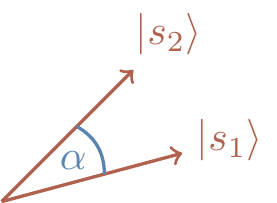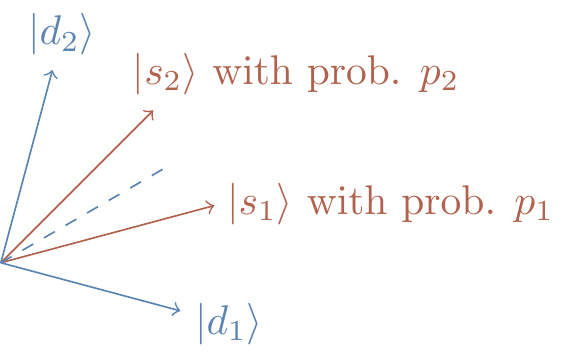## 5.7 Other controlled gates

### 5.7.1 Controlled-phase

Needless to say, not everything is about the controlled-\texttt{NOT} gate. Another common two-qubit gate is the controlled-phase gate, denoted \texttt{c-}P_\varphi.

controlled-phase
\left[\begin{array}{c|c}\begin{matrix}1&0\\0&1\end{matrix}&\begin{matrix}0&0\\0&0\end{matrix}\\\hline\begin{matrix}0&0\\0&0\end{matrix}&\begin{matrix}1&0\\0&e^{i\varphi}\end{matrix}\end{array}\right]

We can also represent the \texttt{c-}P_\varphi gate using the circuit notation, as in Figure 5.2.Figure 5.2: Where x,y\in\{0,1\}.

Again, the matrix is written in the computational basis \{|00\rangle,|01\rangle,|10\rangle,|11\rangle\}. If we do not specify the phase then we usually assume that \varphi=\pi, in which case we call this operation the controlled-Z gate, which acts as |0\rangle\langle 0|\otimes\mathbf{1}+ |1\rangle\langle 1|\otimes Z. Here Z refers again to the Pauli phase-flip \sigma_z\equiv Z operation.

In order to see the entangling power of the controlled-phase shift gate, consider the following circuit.

(Generating entanglement, again).In this circuit, first the two Hadamard gates prepare the equally-weighted superposition of all states from the computational basisand then the controlled-Z operation flips the sign in front of |11\ranglewhich results in an entangled state.

### 5.7.2 Controlled-U

Both the above two-qubit controlled gates (i.e. \texttt{c-NOT} and \texttt{c-}P_\varphi) are specific examples of the more general construction of a controlled-U gate: \texttt{c-}U = |0\rangle\langle 0|\otimes\mathbf{1}+ |1\rangle\langle 1|\otimes U where U is an arbitrary single-qubit unitary transformation U.

controlled-U
\left[\begin{array}{c|c}\begin{matrix}1&0\\0&1\end{matrix}&\begin{matrix}0&0\\0&0\end{matrix}\\\hline\begin{matrix}0&0\\0&0\end{matrix}&U\end{array}\right]

We can also represent the \texttt{c-}U gate using the circuit notation, as in Figure 5.3.Figure 5.3: The controlled-U gate, where x,y\in\{0,1\}.

We can go even further and consider a more general unitary operation: the two-qubit x-controlled-U gate: \sum_x |x\rangle\langle x|\otimes U_x \equiv |0\rangle\langle 0|\otimes U_0 + |1\rangle\langle 1|\otimes U_1 where each U_x is a unitary transformation that is applied to the second qubit only if the first one is in state |x\rangle. In general, an x-controlled-U gate can be defined on two registers of arbitrary size n and m, with x\in\{0,1\}^n and the U_x being (2^m\times 2^m) unitary matrices acting on the second register.

### 5.7.3 Phase kick-back

Before moving on to the next section, we first describe a simple “trick” — an unusual way of introducing phase shifts that will be essential for our analysis of quantum algorithms. Consider the following circuit.

(Controlled-U interference).where |u\rangle is an eigenstate of U, so that U|u\rangle = e^{i\varphi}|u\rangle for some \varphi.

This should look familiar: it is the usual interference circuit, but with the phase gate replaced by a controlled-U gate, which will mimic the phase gate, as we shall soon see. Note that the second qubit is prepared in state |u\rangle, which is required to be an eigenstate of U. The circuit effects the following sequence of transformations:100 \begin{aligned} |0\rangle|u\rangle &\overset{H}{\longmapsto} (|0\rangle+|1\rangle)|u\rangle \\&\quad = |0\rangle|u\rangle + |1\rangle|u\rangle \\&\overset{\texttt{c-}U}{\longmapsto} |0\rangle|u\rangle + |1\rangle U|u\rangle \\&\quad = |0\rangle|u\rangle + e^{i\varphi}|1\rangle|u\rangle \\&\quad = (|0\rangle + e^{i\varphi}|1\rangle) |u\rangle \\&\overset{H}{\longmapsto} \left( \cos\frac{\varphi}{2}|0\rangle - i\sin\frac{\varphi}{2}|1\rangle \right) |u\rangle. \end{aligned} Note that the second qubit does not get entangled with the first one: it remains in its original state |u\rangle. However, the interaction between the two qubits introduces a phase shift on the first qubit. This may look like an unnecessarily complicated way of introducing phase shifts, but, as we shall soon see, this is how quantum computers do it. Here is a preview of things to come.

Consider the following x-controlled-U operation: \left[ \begin{array}{cccc} \mathbf{1}& 0 & 0 & 0 \\0 & \mathbf{1}& 0 & 0 \\0 & 0 & \mathbf{1}& 0 \\0 & 0 & 0 & X \end{array} \right] = \begin{aligned} |00\rangle\langle 00|&\otimes\mathbf{1} \\+\,\,|01\rangle\langle 01|&\otimes\mathbf{1} \\+\,\,|10\rangle\langle 10|&\otimes\mathbf{1} \\+\,\,|11\rangle\langle 11|&\otimes X. \end{aligned} The first register is of size 2 (corresponding to the top-left 2\times 2 block, which is simply the identity matrix), and the second register is of size 1 (corresponding to the two bottom-right 1\times1 blocks, namely an identity matrix and X). If the first register is prepared in state |11\rangle, then the qubit in the second register is flipped (by the Pauli bit-flip X); otherwise, nothing happens.

This unitary operation is a quantum version of the Boolean function evaluation: it corresponds to the Boolean function \begin{aligned} f\colon\{0,1\}^2 &\longrightarrow \{0,1\} \\00&\longmapsto0 \\01&\longmapsto0 \\10&\longmapsto0 \\11&\longmapsto1. \end{aligned} If f(x)=1, then we flip the bit value in the second register (with operation X); if f(x)=0, then we do nothing.

Now, prepare the qubit in the second register in state |0\rangle-|1\rangle, which is an eigenstate of X with eigenvalue e^{\pi i}=-1. So whenever X is applied to the second register, the phase factor -1 appears in front of the corresponding term in the first register. If we prepare the first register in the superposition |00\rangle+|01\rangle+|10\rangle+|11\rangle then the result of applying the above x-controlled-U operation is the entangled state |00\rangle+|01\rangle+|10\rangle-|11\rangle. That is, the phase kick-back mechanism introduces a relative phase in the equally-weighted superposition of all binary strings of length two.

Phase kick-back is how we control quantum interference in quantum computation.

We will return to this topic later on in Section 11.3, when we discuss quantum evaluation of Boolean functions and quantum algorithms.

### 5.7.4 Universality, revisited

We will come across few more gates in this course, but at this stage you already know all the elementary unitary operations that are needed to construct any unitary operation on any number of qubits:

• all phase gates, and
• the \texttt{c-NOT}

These gates form a universal set of gates: with O(4^{n}n) of these gates, we can construct any n-qubit unitary operation.101 We should mention that there are many different universal sets of gates. In fact, almost any gate that can entangle two qubits can be used as a universal gate.

We are particularly interested in any finite universal set of gates (such as the one containing the Hadamard, P_{\frac{\pi}{4}} (the T gate), and the \texttt{c-NOT}) that can approximate any unitary operation on n qubits with arbitrary precision. The price to pay is the number of gates — better precision requires more gates.

### 5.7.5 Density operators, and other things to come

The existence of entangled states leads to an obvious question: if we cannot attribute a state vector to an individual qubit, then how can we describe its quantum state? In the next few chapters we will see that, when we limit our attentions to a part of a larger system, states are not represented by vectors, measurements are not described by orthogonal projections, and evolution is not unitary. As a spoiler, here is a dictionary of some of the new concepts that will soon be introduced:

 state vectors \rightsquigarrow density operators orthogonal projectors \rightsquigarrow positive operator-valued measures unitary evolutions \rightsquigarrow completely-positive trace-preserving maps

1. Omitting, as per usual, the normalisation factors.↩︎

2. Recall the big-O asymptotic notation introduced in Exercise 1.10.7: given a positive function f(n), we write O(f(n)) to mean “bounded above by c\,f(n) for some constant c > 0 (for sufficiently large n)”. For example, 15n^2+4n+7 is O(n^2).↩︎